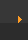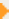## Stoichiometry: Gram Formula Mass (1 of 4)

### Some Notes about Gram Formula Mass (GFM)

• The GFM is also called Molar Mass, Molecular Weight, and Formula Mass. Sometimes it's called Formula Weight. They all mean the same thing.
• The GAM is the mass of one mole of a single element. The GFM is the mass of one mole of all the elements in the molecule or compound (hey, what's the difference between a molecule and a compound?)
• Calculating the Gram Formula Mass is considered a basic skill in chemistry. You need to be able to do it without thinking too hard.
Practice: Gram Formula Mass
 Next pageGFM stands for Gram Formula Mass and describes the mass of one mole of a compound.

To find the GFM, add the individual GAM for each element in the compound (e.g. H2O = 1.01 +1.01 + 16.00 = 18.02 g/mol).

The subscript following an element symbol indicates the number of that element (e.g. H20 tells you there are two H and one O).

The units for the GFM are grams/mole or g/mol.

The Gram Formula Mass is also called the Molar Mass and Molecular Weight.